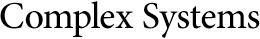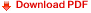## A Cellular Automaton for a Solvable Boltzmann EquationHans Hersbach
Institute for Theoretical Physics, University of Utrecht,
P.O. Box 80006, 3508 TA Utrecht, The Netherlands

#### Abstract

A probabilistic cellular automaton as an approximation of a solvable Boltzmann equation in one space and one time dimension is presented. First the model that was introduced by Ruijgrok and Wu [6,7] is revised in a Lorentz covariant form. Next, an appropriate cellular automaton is defined. By analogy with the Boltzmann approach, equations for the time dependence of ensemble averages of single-particle quantities are obtained by making the Stosszahlansatz. The required nonlinearity is a consequence of the exclusion principle. In accordance with the Boltzmann equation, homogeneous as well as inhomogeneous equilibria are derived exactly. On the basis of intuition and symmetry considerations, a relationship between the two systems is found. By means of this relationship it is demonstrated that the ensemble averaged automaton approximates the Ruijgrok--Wu model up to first order of the lattice spacing, for all time scales. Simulations show that the Stosszahlansatz is justified.